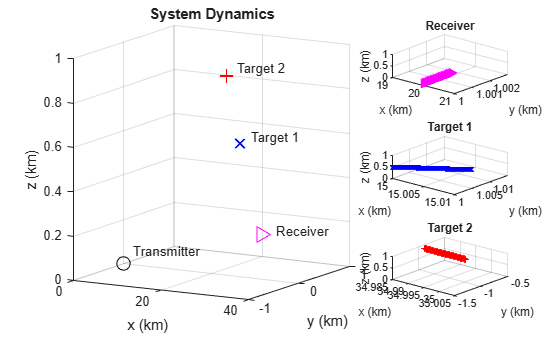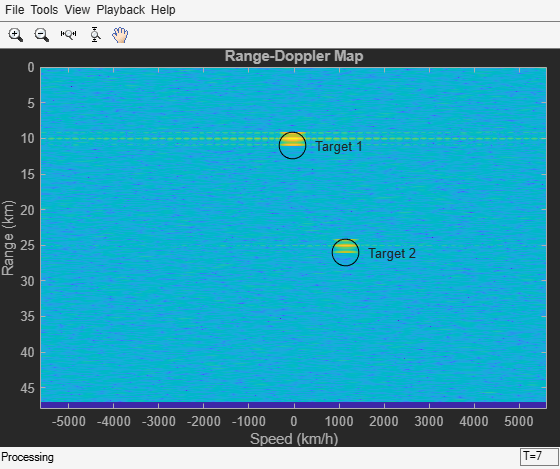Documentation

## Simulating a Bistatic Polarimetric Radar

This example shows how to simulate a polarimetric bistatic radar system to estimate the range and speed of targets. Transmitter, receiver and target kinematics are taken into account. For more information regarding polarization modeling capabilities, see Modeling and Analyzing Polarization.

### System Setup

The system operates at 300 MHz, using a linear FM waveform whose maximum unambiguous range is 48 km. The range resolution is 50 meters and the time-bandwidth product is 20.

```maxrng = 48e3; % Maximum range rngres = 50; % Range resolution tbprod = 20; % Time-bandwidth product ```

The transmitter has a peak power of 2 kw and a gain of 20 dB. The receiver also provides a gain of 20 dB and the noise bandwidth is the same as the waveform's sweep bandwidth.

The transmit antenna array is a stationary 4-element ULA located at origin. The array is made of vertical dipoles.

```txAntenna = phased.ShortDipoleAntennaElement('AxisDirection','Z'); [waveform,transmitter,txmotion,radiator] = ... helperBistatTxSetup(maxrng,rngres,tbprod,txAntenna); ```

The receive antenna array is also a 4-element ULA; it is located at [20000;1000;100] meters away from the transmit antenna and is moving at a velocity of [0;20;0] m/s. Assume the elements in the receive array are also vertical dipoles. The received antenna array is oriented so that its broadside points back to the transmit antenna.

```rxAntenna = phased.ShortDipoleAntennaElement('AxisDirection','Z'); [collector,receiver,rxmotion,rngdopresp,beamformer] = ... helperBistatRxSetup(rngres,rxAntenna); ```

There are two targets present in space. The first one is a point target modeled as a sphere; it preserves the polarization state of the incident signal. It is located at [15000;1000;500] meters away from the transmit array and is moving at a velocity of [100;100;0] m/s.

The second target is located at [35000;-1000;1000] meters away from the transmit array and is approaching at a velocity of [-160;0;-50] m/s. Unlike the first target, the second target flips the polarization state of the incident signal, which means that the horizontal/vertical polarization components of the input signal becomes the vertical/horizontal polarization components of the output signal.

```[target,tgtmotion,txchannel,rxchannel] = ... helperBistatTargetSetup(waveform.SampleRate); ```

A single scattering matrix is a fairly simple polarimetric model for a target. It assumes that no matter what the incident and reflecting directions are, the power distribution between the H and V components is fixed. However, even such a simple model can reveal complicated target behavior in the simulation because (1) the H and V directions vary for different incident and reflecting directions; and (2) the orientation, defined by the local coordinate system, of the targets also affects the polarization matching.

### System Simulation

Next section simulates 256 received pulses. The receiving array is beamformed toward the two targets. The first figure shows the system setting and how the receive array and the targets move. The second figure shows a range-Doppler map generated for every 64 pulses received at the receiver array.

```Nblock = 64; % Burst size dt = 1/waveform.PRF; y = complex(zeros(round(waveform.SampleRate*dt),Nblock)); hPlots = helperBistatViewSetup(txmotion,rxmotion,tgtmotion,waveform,... rngdopresp,y); Npulse = Nblock*4; for m = 1:Npulse % Update positions of transmitter, receiver, and targets [tpos,tvel,txax] = txmotion(dt); [rpos,rvel,rxax] = rxmotion(dt); [tgtp,tgtv,tgtax] = tgtmotion(dt); % Calculate the target angles as seen by the transmitter [txrng,radang] = rangeangle(tgtp,tpos,txax); % Simulate propagation of pulse in direction of targets wav = waveform(); wav = transmitter(wav); sigtx = radiator(wav,radang,txax); sigtx = txchannel(sigtx,tpos,tgtp,tvel,tgtv); % Reflect pulse off of targets for n = 2:-1:1 % Calculate bistatic forward and backward angles for each target [~,fwang] = rangeangle(tpos,tgtp(:,n),tgtax(:,:,n)); [rxrng(n),bckang] = rangeangle(rpos,tgtp(:,n),tgtax(:,:,n)); sigtgt(n) = target{n}(sigtx(n),fwang,bckang,tgtax(:,:,n)); end % Receive path propagation sigrx = rxchannel(sigtgt,tgtp,rpos,tgtv,rvel); [~,inang] = rangeangle(tgtp,rpos,rxax); rspeed_t = radialspeed(tgtp,tgtv,tpos,tvel); rspeed_r = radialspeed(tgtp,tgtv,rpos,rvel); % Receive target returns at bistatic receiver sigrx = collector(sigrx,inang,rxax); yc = beamformer(sigrx,inang); y(:,mod(m-1,Nblock)+1) = receiver(sum(yc,2)); helperBistatViewTrajectory(hPlots,tpos,rpos,tgtp); if ~rem(m,Nblock) rd_rng = (txrng+rxrng)/2; rd_speed = rspeed_t+rspeed_r; helperBistatViewSignal(hPlots,waveform,rngdopresp,y,rd_rng,... rd_speed) end end ```The Range-Doppler map only shows the return from the first target. This is probably no surprise since both the transmit and receive array are vertically polarized and the second target maps the vertically polarized wave to horizontally polarized wave. The received signal from the second target is mostly orthogonal to the receive array's polarization, resulting in significant polarization loss.

One may also notice that the resulting range and radial speed do not agree with the range and radial speed of the target relative to the transmitter. This is because in a bistatic configuration, the estimated range is actually the geometric mean of the target range relative to the transmitter and the receiver. Similarly, the estimated radial speed is the sum of the target radial speed relative to the transmitter and the receiver. The circle in the map shows where the targets should appear in the range-Doppler map. Further processing is required to identify the exact location of the target, but those are beyond the scope of this example.

### Using Circularly Polarized Receive Array

Vertical dipole is a very popular choice of transmit antenna in real applications because it is low cost and have a omnidirectional pattern. However, the previous simulation shows that if the same antenna is used in the receiver, there is a risk that the system will miss certain targets. Therefore, a linear polarized antenna is often not the best choice as the receive antenna in such a configuration because no matter how the linear polarization is aligned, there always exists an orthogonal polarization. In case the reflected signal bears a polarization state close to that direction, the polarization loss becomes huge.

One way to solve this issue is to use a circularly polarized antenna at the receive end. A circularly polarized antenna cannot fully match any linear polarization. But on the other hand, the polarization loss between a circular polarized antenna and a linearly polarized signal is 3 dB, regardless what direction the linear polarization is in. Therefore, although it never gives the maximum return, it never misses a target. A frequently used antenna with circular polarization is a crossed dipole antenna.

Next section shows what happens when crossed dipole antennas are used to form the receive array.

```rxAntenna = phased.CrossedDipoleAntennaElement; collector = clone(collector); collector.Sensor.Element = rxAntenna; helperBistatSystemRun(waveform,transmitter,txmotion,radiator,collector,... receiver,rxmotion,rngdopresp,beamformer,target,tgtmotion,txchannel,... rxchannel,hPlots,Nblock,Npulse); ```The range-Doppler map now shows both targets at their correct locations.

### Summary

This example shows a system level simulation of a bistatic polarimetric radar. The example generates range-Doppler maps of the received signal for different transmit/receive array polarization configurations and shows how a circularly polarized antenna can be used to avoid losing linear polarized signals due to a target's polarization scattering property.